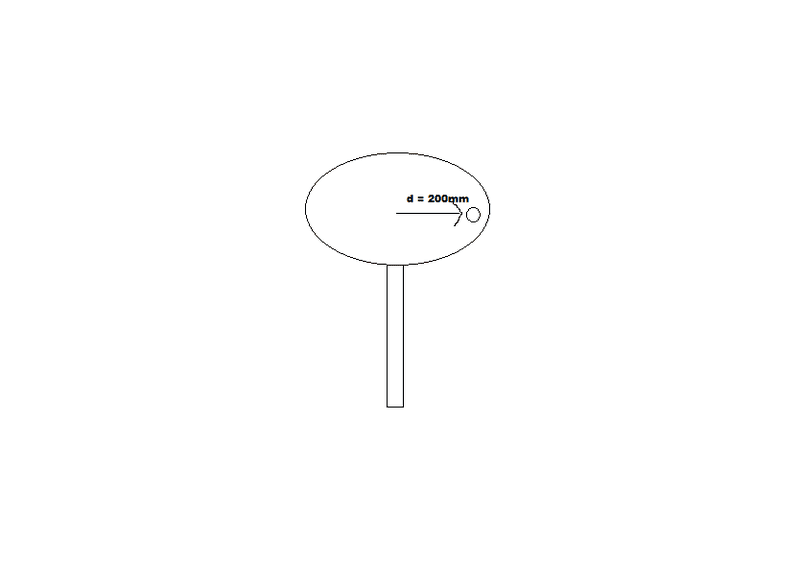# Angular velocity and acceleration

• Nikstykal

## Homework Statement

Small mass sits on a circular revolving table, 200 mm from center. It is given a constant angular acceleration of 2 rad/s. The static coefficient of friction is 0.2. At what angular velocity will the mass start to slip?ar=v2/r
ar=r''-rθ'2
aθ=rθ''+2r'θ'
ΣFi=mai
Ff,max=μN

## The Attempt at a Solution

I first set up a free body diagram with wmass pointing downwards, Ff pointing left, normal pointing upwards, with ar vector pointing to the right.

Since the distance is unchanging, r = 0.2m, r'=0, r''=0. θ''=2rad/s, θ'=2t rad/s + c, θ=t2+ct+d

ΣFz=maz=N-mg, since az=0, N=mg
ΣFr=mar=m(r''-rθ'2)=-Ff
Combining: m(r''-rθ'2)=-μmg
Simplifying: -rθ'2=-μg

θ' = ω = √(μg/r)
θ' = √(0.2⋅(9.81m/s2)/0.2m) = 3.13 rad/s

Does this look correct? I never used the given angular acceleration so I feel like I missed something. Thank you.